# 14 PARTIAL DERIVATIVES PARTIAL DERIVATIVES 14 2 Limits

• Slides: 9414 PARTIAL DERIVATIVES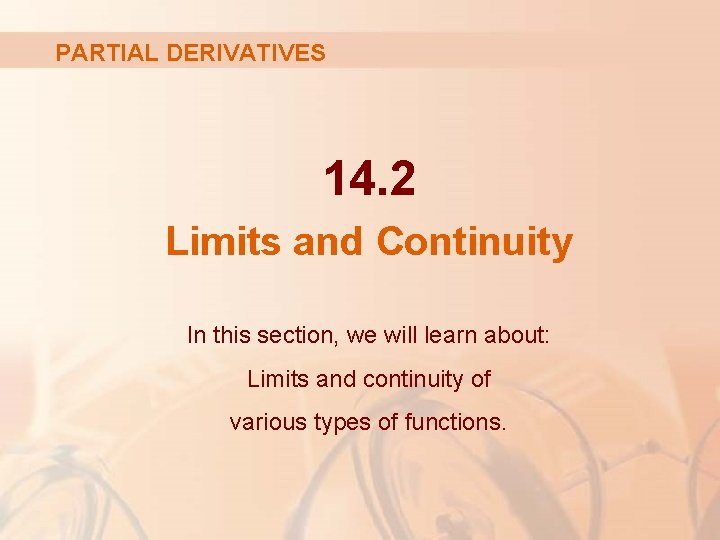PARTIAL DERIVATIVES 14. 2 Limits and Continuity In this section, we will learn about: Limits and continuity of various types of functions.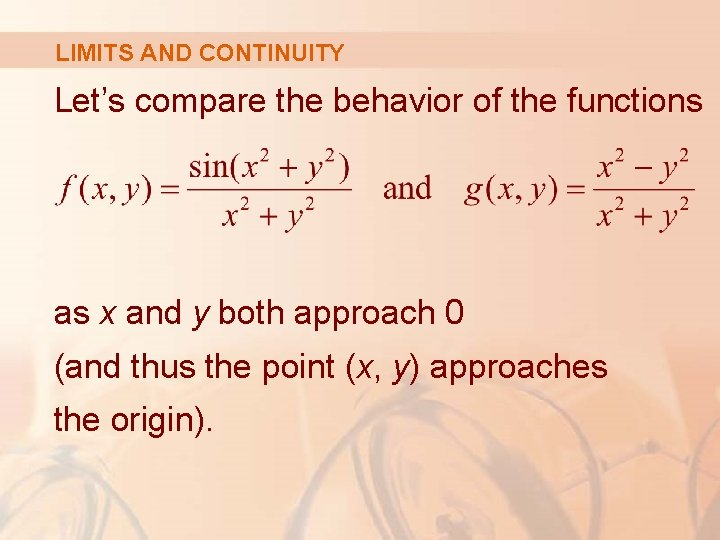LIMITS AND CONTINUITY Let’s compare the behavior of the functions as x and y both approach 0 (and thus the point (x, y) approaches the origin).LIMITS AND CONTINUITY The following tables show values of f(x, y) and g(x, y), correct to three decimal places, for points (x, y) near the origin.LIMITS AND CONTINUITY Table 1 This table shows values of f(x, y).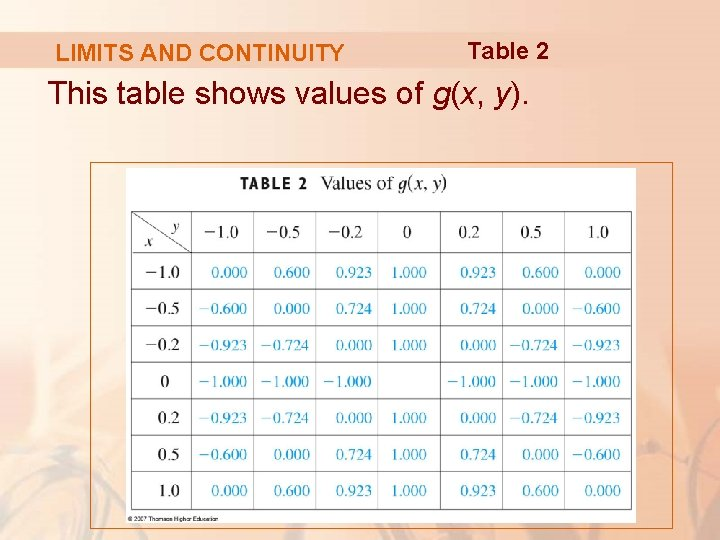LIMITS AND CONTINUITY Table 2 This table shows values of g(x, y).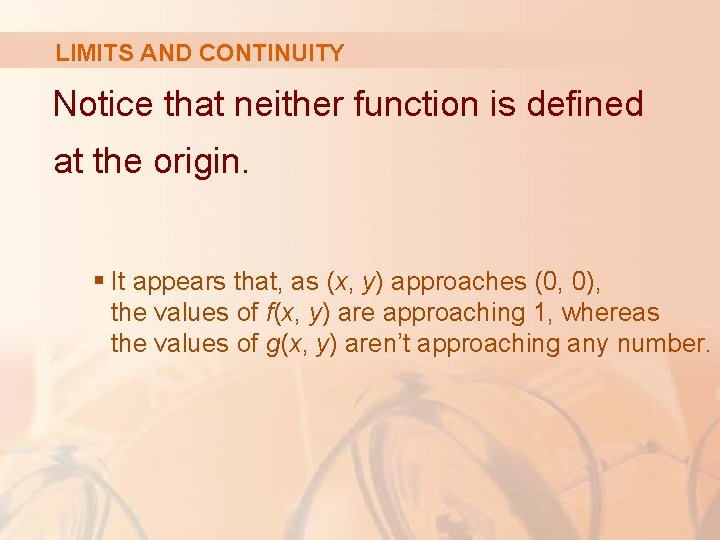LIMITS AND CONTINUITY Notice that neither function is defined at the origin. § It appears that, as (x, y) approaches (0, 0), the values of f(x, y) are approaching 1, whereas the values of g(x, y) aren’t approaching any number.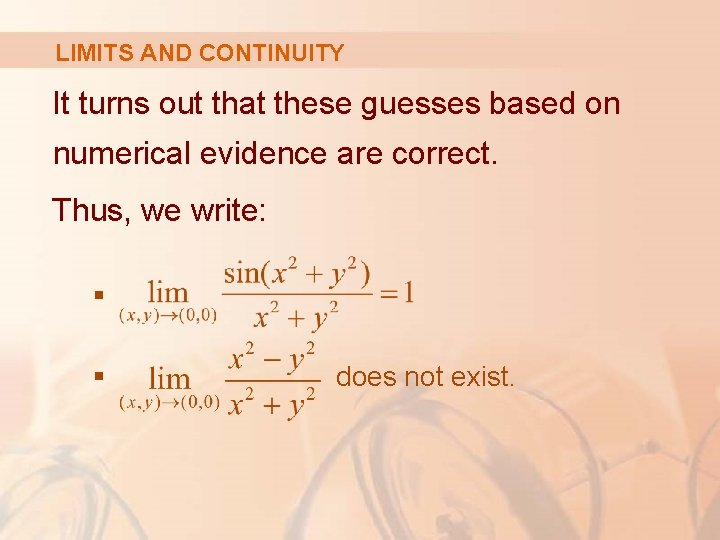LIMITS AND CONTINUITY It turns out that these guesses based on numerical evidence are correct. Thus, we write: § § does not exist.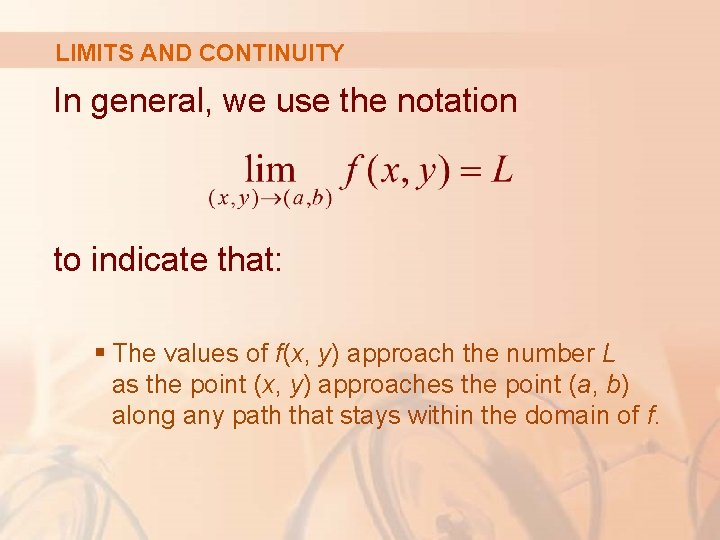LIMITS AND CONTINUITY In general, we use the notation to indicate that: § The values of f(x, y) approach the number L as the point (x, y) approaches the point (a, b) along any path that stays within the domain of f.LIMITS AND CONTINUITY In other words, we can make the values of f(x, y) as close to L as we like by taking the point (x, y) sufficiently close to the point (a, b), but not equal to (a, b). § A more precise definition follows.LIMIT OF A FUNCTION Definition 1 Let f be a function of two variables whose domain D includes points arbitrarily close to (a, b). Then, we say that the limit of f(x, y) as (x, y) approaches (a, b) is L.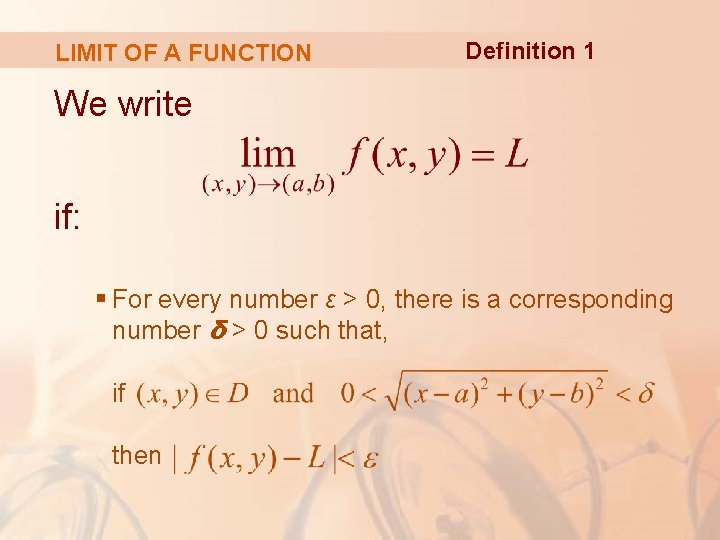LIMIT OF A FUNCTION Definition 1 We write if: § For every number ε > 0, there is a corresponding number δ > 0 such that, if then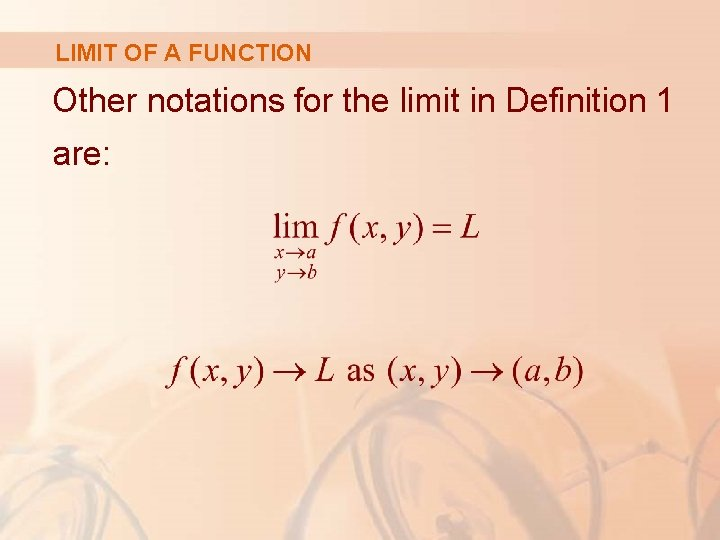LIMIT OF A FUNCTION Other notations for the limit in Definition 1 are:LIMIT OF A FUNCTION Notice that: § is the distance between the numbers f(x, y) and L § is the distance between the point (x, y) and the point (a, b).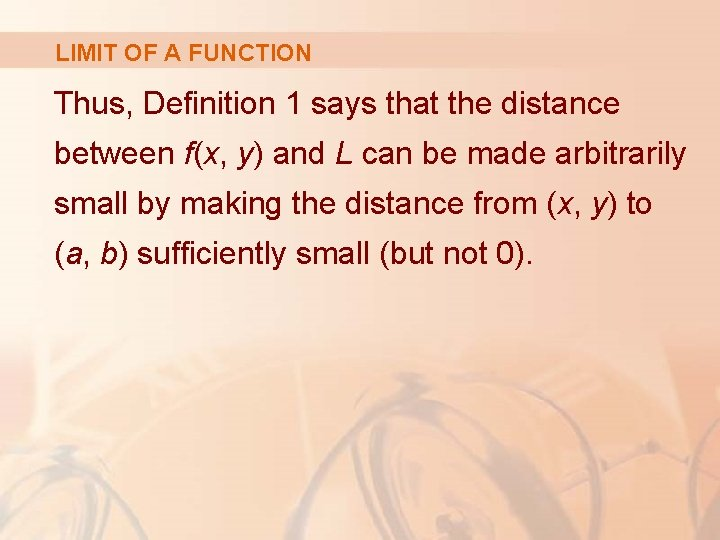LIMIT OF A FUNCTION Thus, Definition 1 says that the distance between f(x, y) and L can be made arbitrarily small by making the distance from (x, y) to (a, b) sufficiently small (but not 0).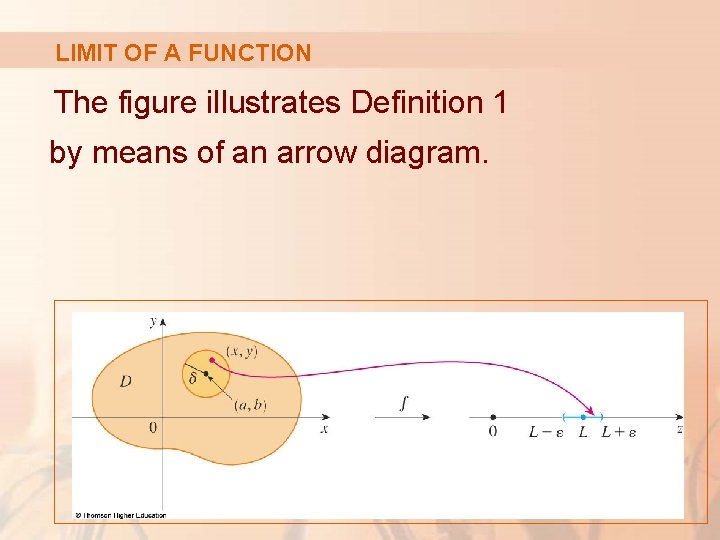LIMIT OF A FUNCTION The figure illustrates Definition 1 by means of an arrow diagram.LIMIT OF A FUNCTION If any small interval (L – ε, L + ε) is given around L, then we can find a disk Dδ with center (a, b) and radius δ > 0 such that: § f maps all the points in Dδ [except possibly (a, b)] into the interval (L – ε, L + ε).LIMIT OF A FUNCTION Another illustration of Definition 1 is given here, where the surface S is the graph of f.LIMIT OF A FUNCTION If ε > 0 is given, we can find δ > 0 such that, if (x, y) is restricted to lie in the disk Dδ and (x, y) ≠ (a, b), then § The corresponding part of S lies between the horizontal planes z = L – ε and z = L + ε.SINGLE VARIABLE FUNCTIONS For functions of a single variable, when we let x approach a, there are only two possible directions of approach, from the left or from the right. § We recall from Chapter 2 that, if then does not exist.DOUBLE VARIABLE FUNCTIONS For functions of two variables, the situation is not as simple.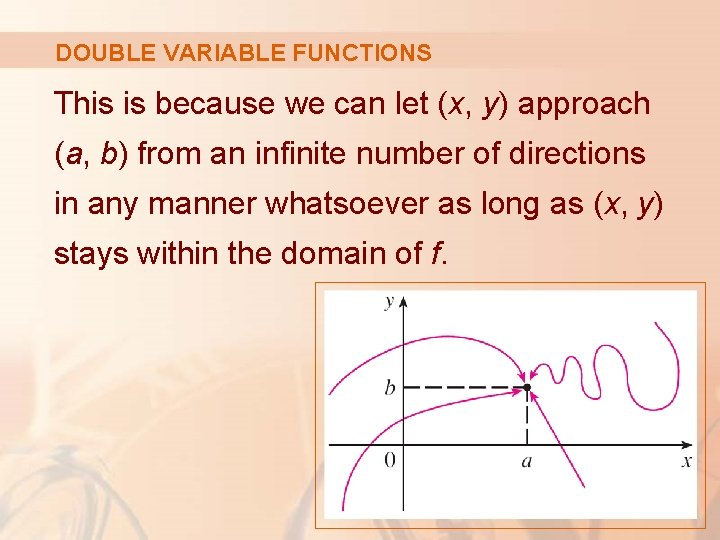DOUBLE VARIABLE FUNCTIONS This is because we can let (x, y) approach (a, b) from an infinite number of directions in any manner whatsoever as long as (x, y) stays within the domain of f.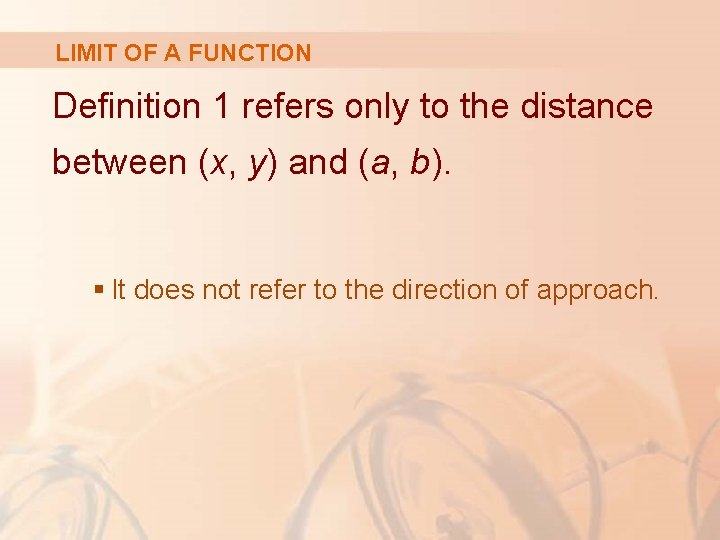LIMIT OF A FUNCTION Definition 1 refers only to the distance between (x, y) and (a, b). § It does not refer to the direction of approach.LIMIT OF A FUNCTION Therefore, if the limit exists, then f(x, y) must approach the same limit no matter how (x, y) approaches (a, b).LIMIT OF A FUNCTION Thus, if we can find two different paths of approach along which the function f(x, y) has different limits, then it follows that does not exist.LIMIT OF A FUNCTION If f(x, y) → L 1 as (x, y) → (a, b) along a path C 1 and f(x, y) → L 2 as (x, y) → (a, b) along a path C 2, where L 1 ≠ L 2, then does not exist.LIMIT OF A FUNCTION Example 1 Show that does not exist. § Let f(x, y) = (x 2 – y 2)/(x 2 + y 2).LIMIT OF A FUNCTION Example 1 First, let’s approach (0, 0) along the x-axis. § Then, y = 0 gives f(x, 0) = x 2/x 2 = 1 for all x ≠ 0. § So, f(x, y) → 1 as (x, y) → (0, 0) along the x-axis.LIMIT OF A FUNCTION Example 1 We now approach along the y-axis by putting x = 0. § Then, f(0, y) = –y 2/y 2 = – 1 for all y ≠ 0. § So, f(x, y) → – 1 as (x, y) → (0, 0) along the y-axis.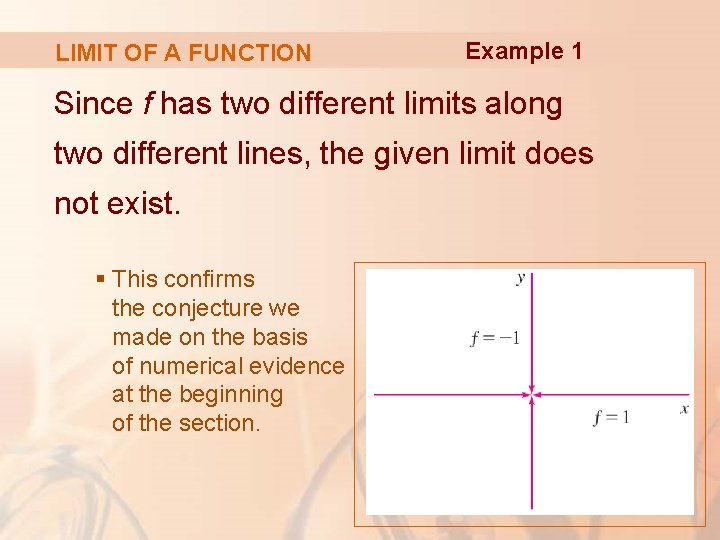LIMIT OF A FUNCTION Example 1 Since f has two different limits along two different lines, the given limit does not exist. § This confirms the conjecture we made on the basis of numerical evidence at the beginning of the section.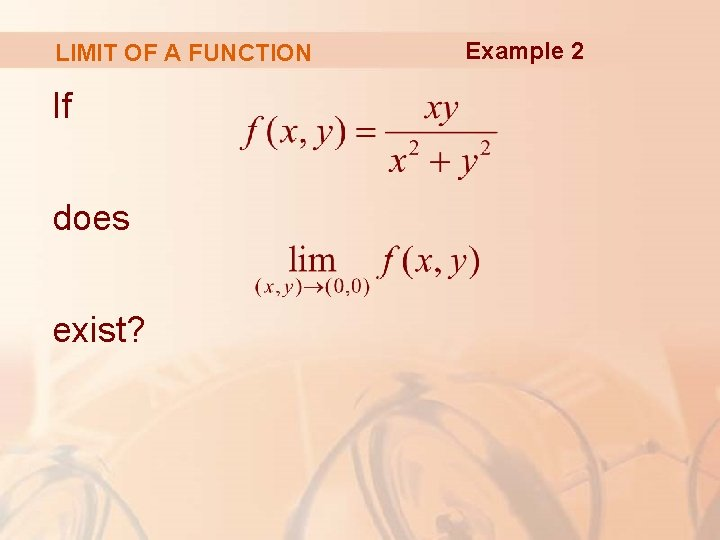LIMIT OF A FUNCTION If does exist? Example 2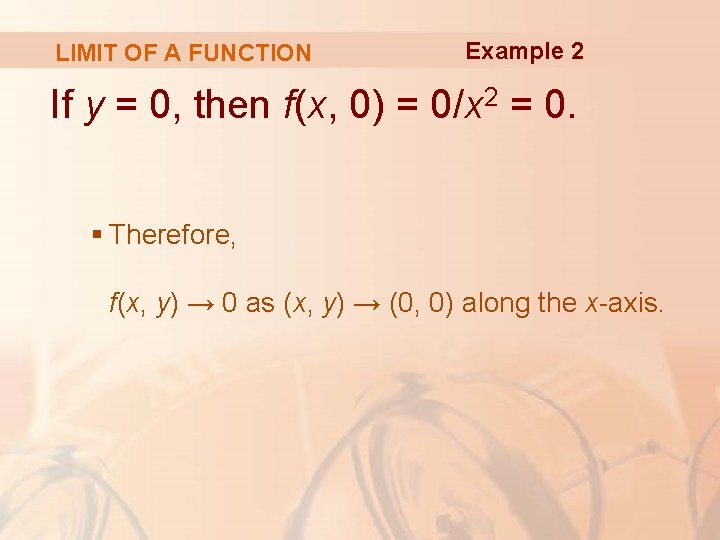LIMIT OF A FUNCTION Example 2 If y = 0, then f(x, 0) = 0/x 2 = 0. § Therefore, f(x, y) → 0 as (x, y) → (0, 0) along the x-axis.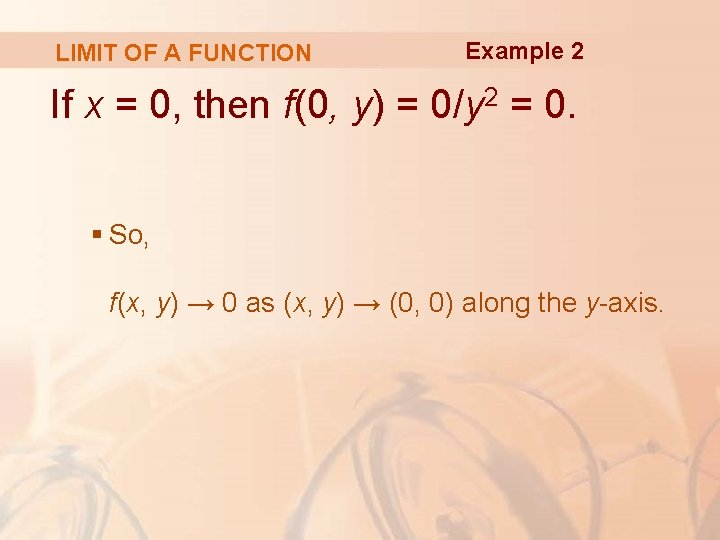LIMIT OF A FUNCTION Example 2 If x = 0, then f(0, y) = 0/y 2 = 0. § So, f(x, y) → 0 as (x, y) → (0, 0) along the y-axis.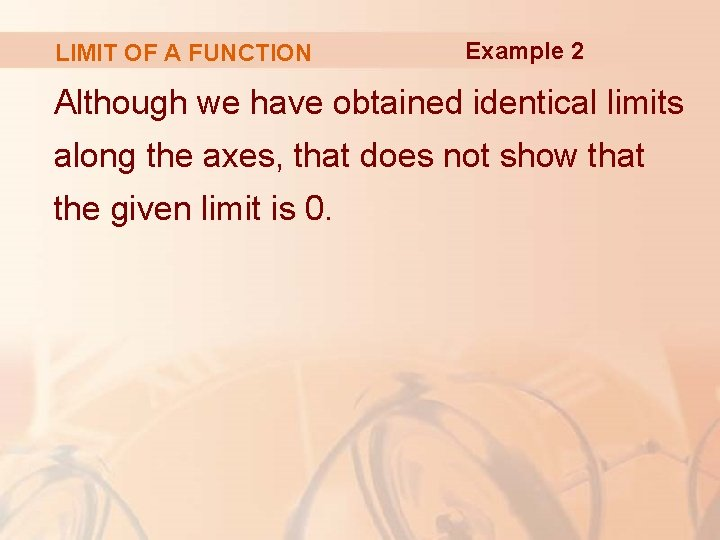LIMIT OF A FUNCTION Example 2 Although we have obtained identical limits along the axes, that does not show that the given limit is 0.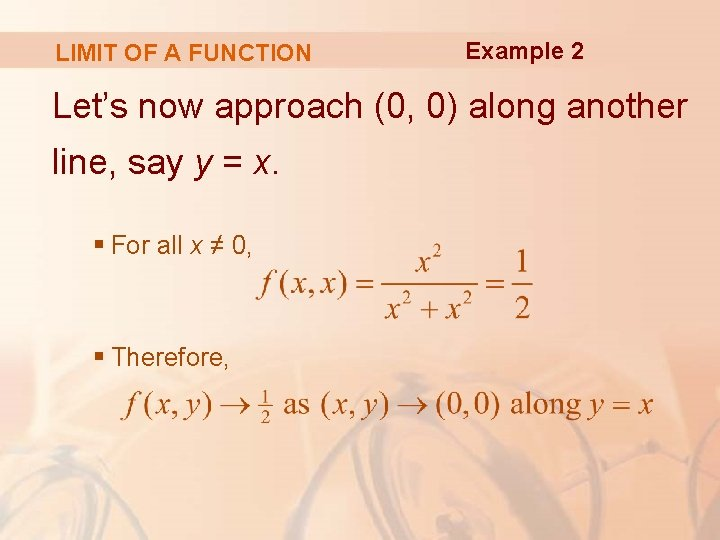LIMIT OF A FUNCTION Example 2 Let’s now approach (0, 0) along another line, say y = x. § For all x ≠ 0, § Therefore,LIMIT OF A FUNCTION Example 2 Since we have obtained different limits along different paths, the given limit does not exist.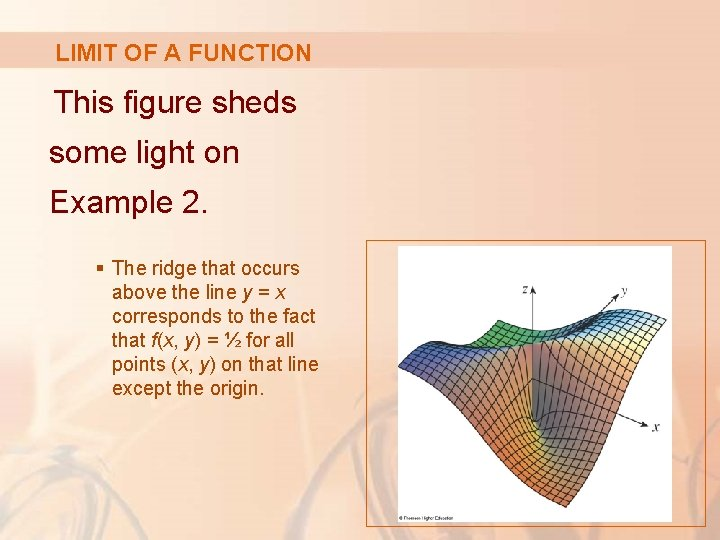LIMIT OF A FUNCTION This figure sheds some light on Example 2. § The ridge that occurs above the line y = x corresponds to the fact that f(x, y) = ½ for all points (x, y) on that line except the origin.LIMIT OF A FUNCTION If does exist? Example 3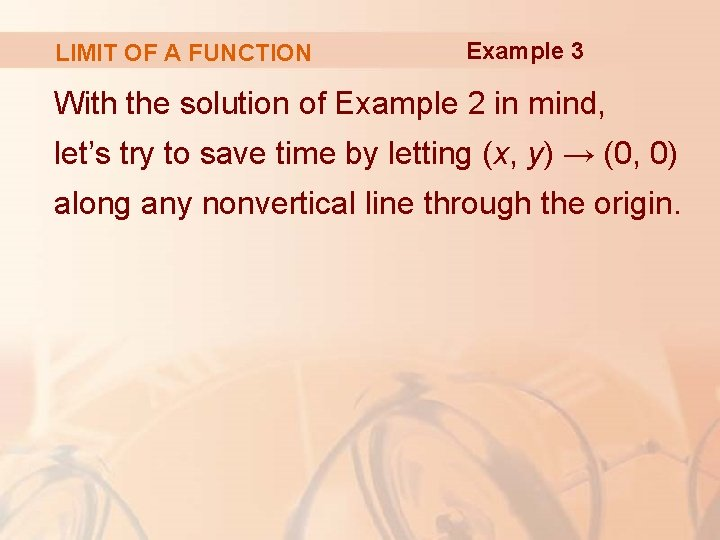LIMIT OF A FUNCTION Example 3 With the solution of Example 2 in mind, let’s try to save time by letting (x, y) → (0, 0) along any nonvertical line through the origin.LIMIT OF A FUNCTION Example 3 Then, y = mx, where m is the slope, and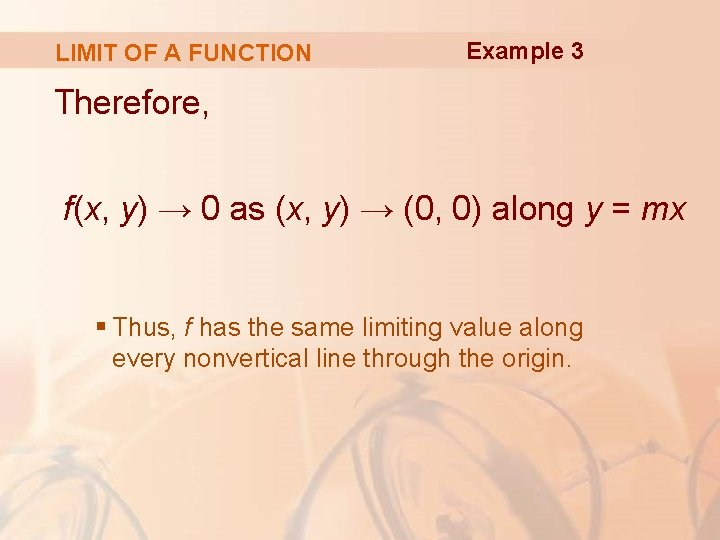LIMIT OF A FUNCTION Example 3 Therefore, f(x, y) → 0 as (x, y) → (0, 0) along y = mx § Thus, f has the same limiting value along every nonvertical line through the origin.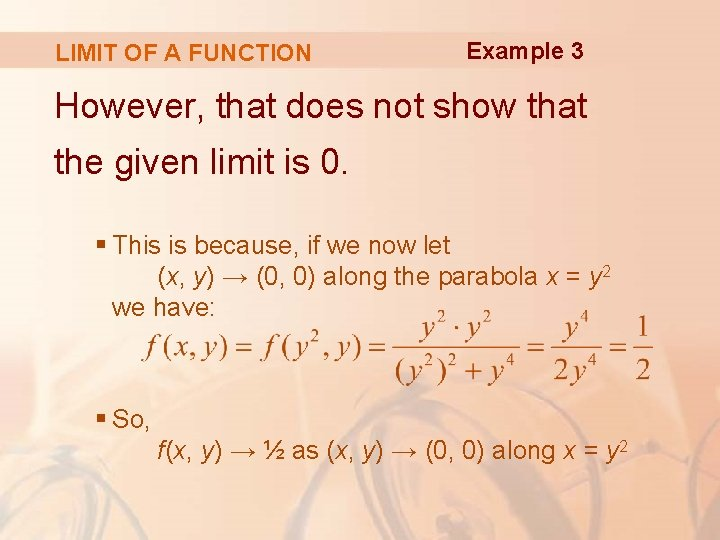LIMIT OF A FUNCTION Example 3 However, that does not show that the given limit is 0. § This is because, if we now let (x, y) → (0, 0) along the parabola x = y 2 we have: § So, f(x, y) → ½ as (x, y) → (0, 0) along x = y 2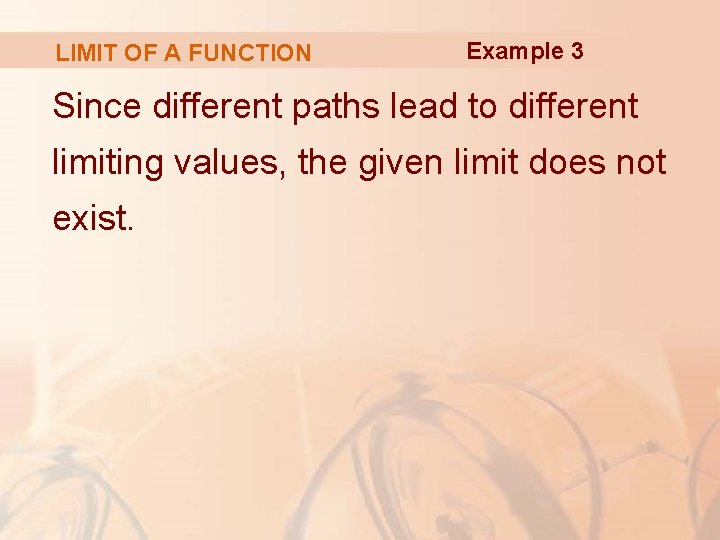LIMIT OF A FUNCTION Example 3 Since different paths lead to different limiting values, the given limit does not exist.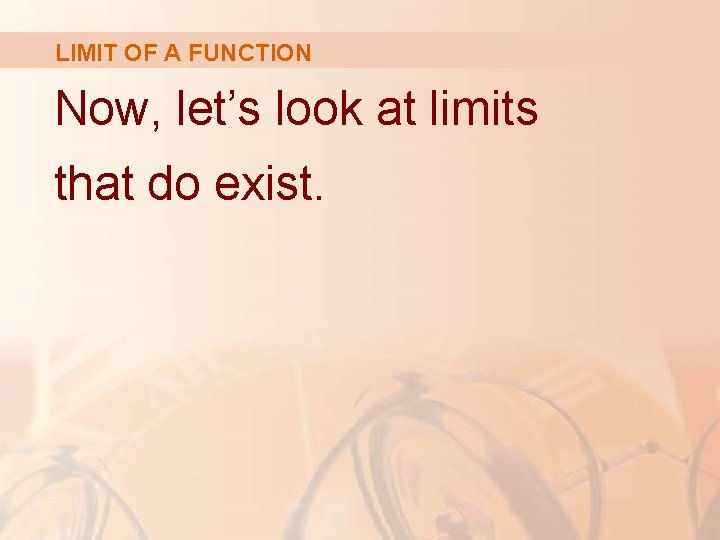LIMIT OF A FUNCTION Now, let’s look at limits that do exist.LIMIT OF A FUNCTION Just as for functions of one variable, the calculation of limits for functions of two variables can be greatly simplified by the use of properties of limits.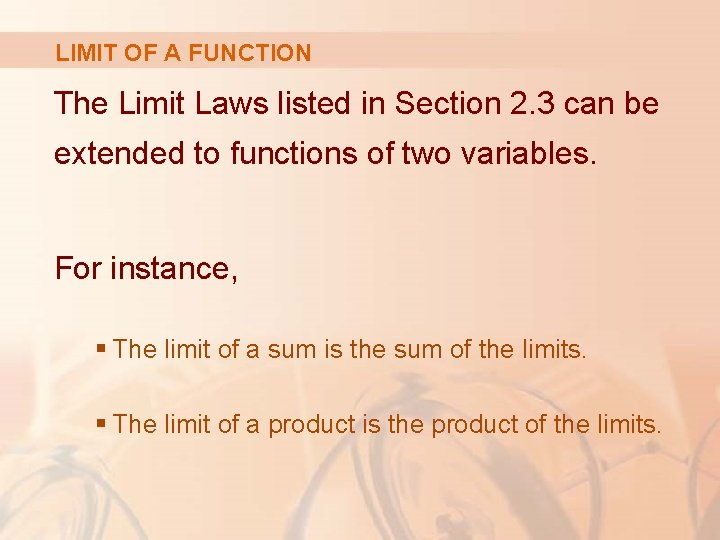LIMIT OF A FUNCTION The Limit Laws listed in Section 2. 3 can be extended to functions of two variables. For instance, § The limit of a sum is the sum of the limits. § The limit of a product is the product of the limits.LIMIT OF A FUNCTION Equations 2 In particular, the following equations are true.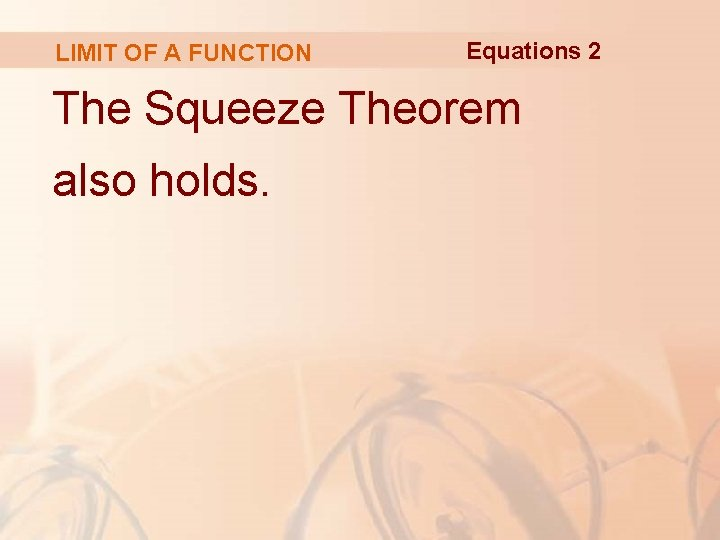LIMIT OF A FUNCTION Equations 2 The Squeeze Theorem also holds.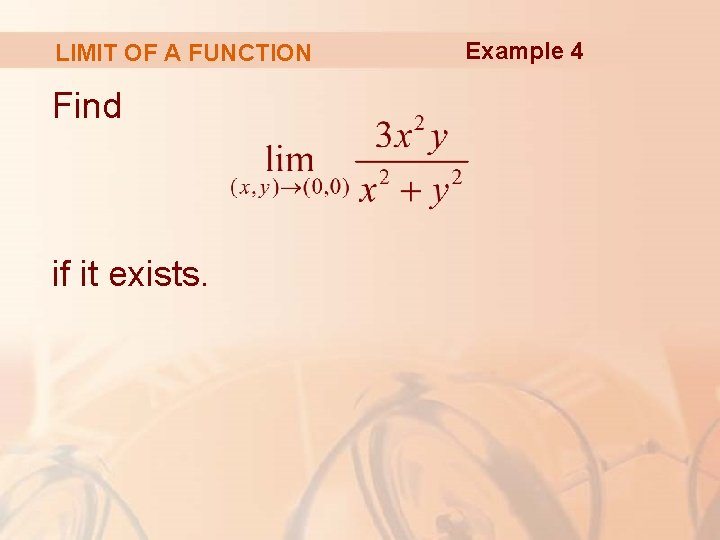LIMIT OF A FUNCTION Find if it exists. Example 4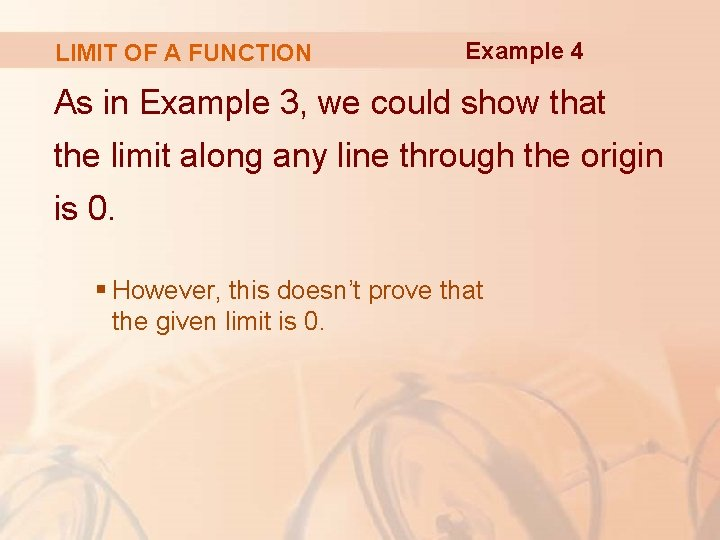LIMIT OF A FUNCTION Example 4 As in Example 3, we could show that the limit along any line through the origin is 0. § However, this doesn’t prove that the given limit is 0.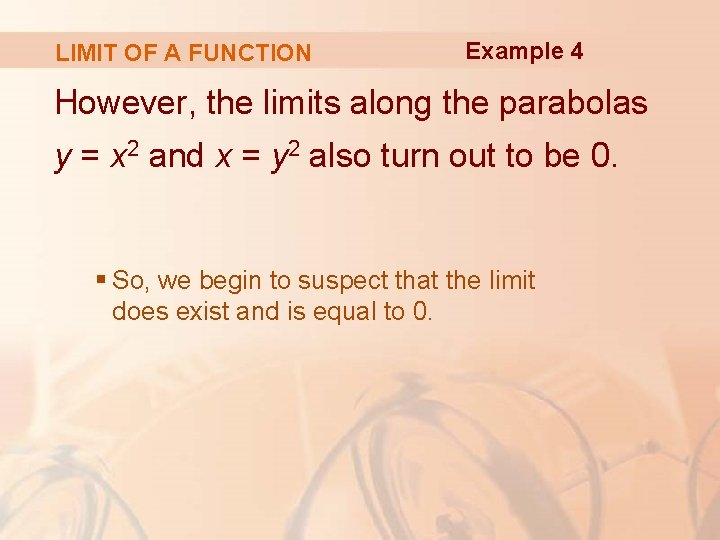LIMIT OF A FUNCTION Example 4 However, the limits along the parabolas y = x 2 and x = y 2 also turn out to be 0. § So, we begin to suspect that the limit does exist and is equal to 0.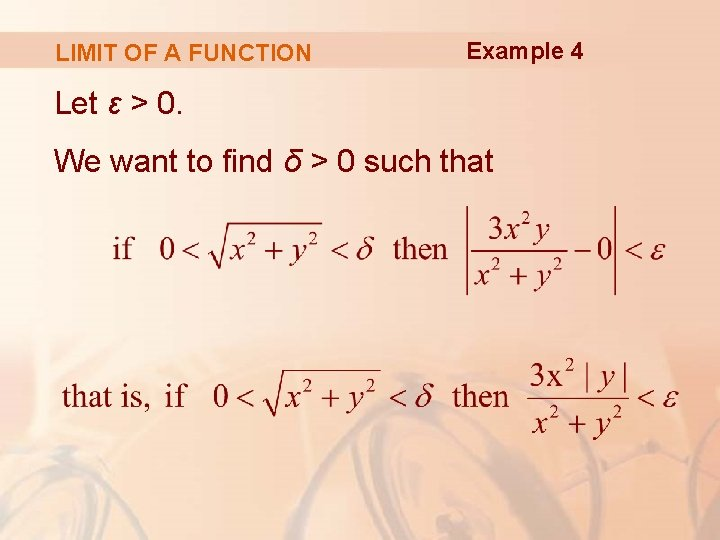LIMIT OF A FUNCTION Example 4 Let ε > 0. We want to find δ > 0 such that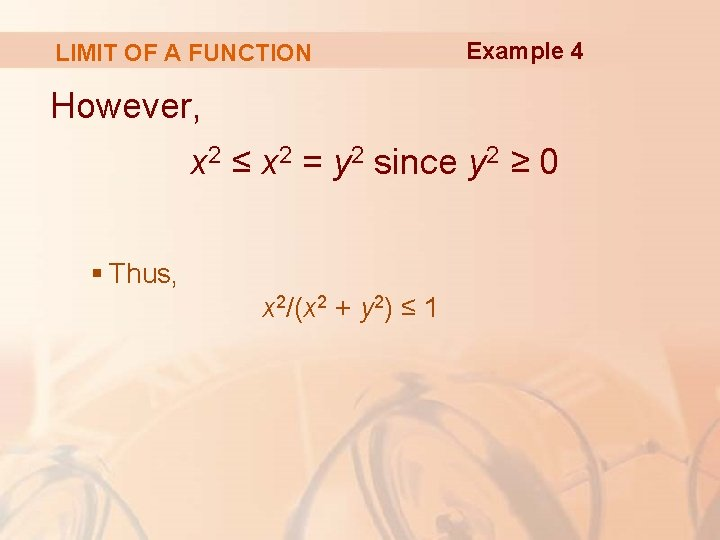LIMIT OF A FUNCTION Example 4 However, x 2 ≤ x 2 = y 2 since y 2 ≥ 0 § Thus, x 2/(x 2 + y 2) ≤ 1LIMIT OF A FUNCTION Therefore, E. g. 4—Equation 3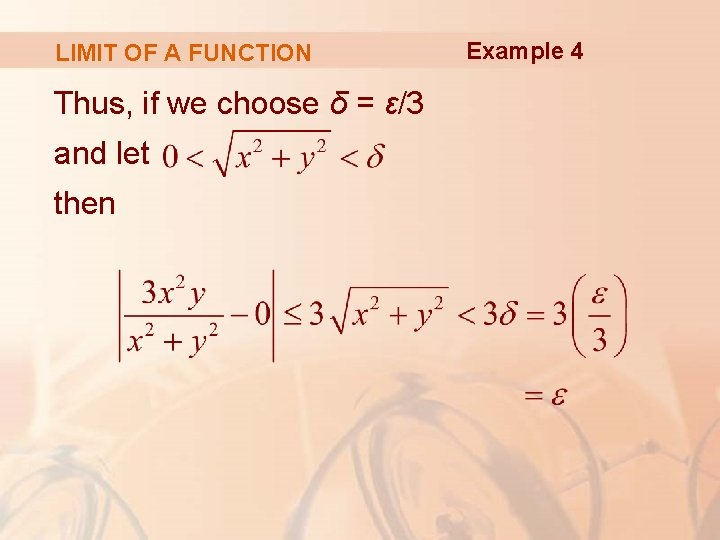LIMIT OF A FUNCTION Thus, if we choose δ = ε/3 and let then Example 4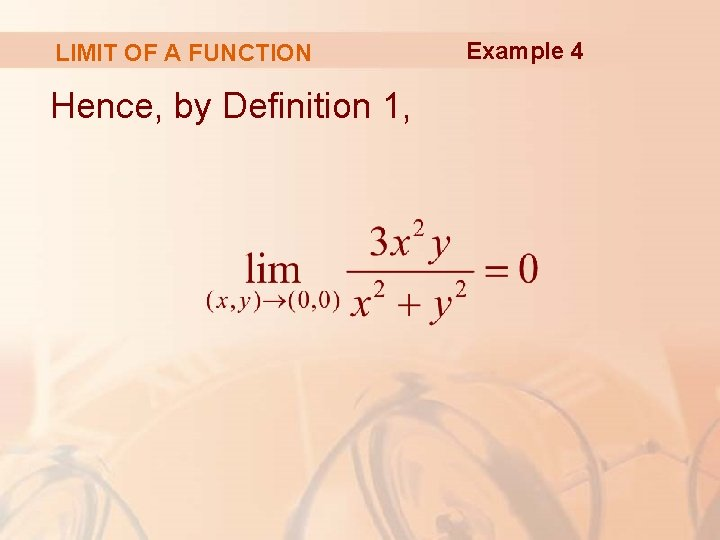LIMIT OF A FUNCTION Hence, by Definition 1, Example 4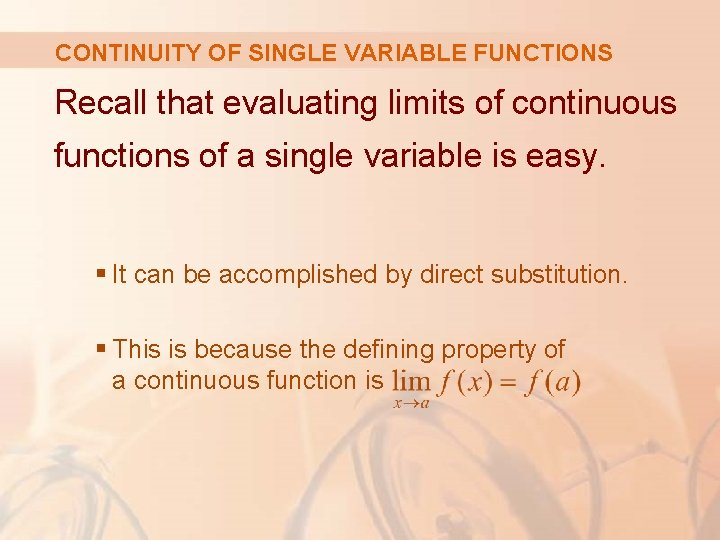CONTINUITY OF SINGLE VARIABLE FUNCTIONS Recall that evaluating limits of continuous functions of a single variable is easy. § It can be accomplished by direct substitution. § This is because the defining property of a continuous function is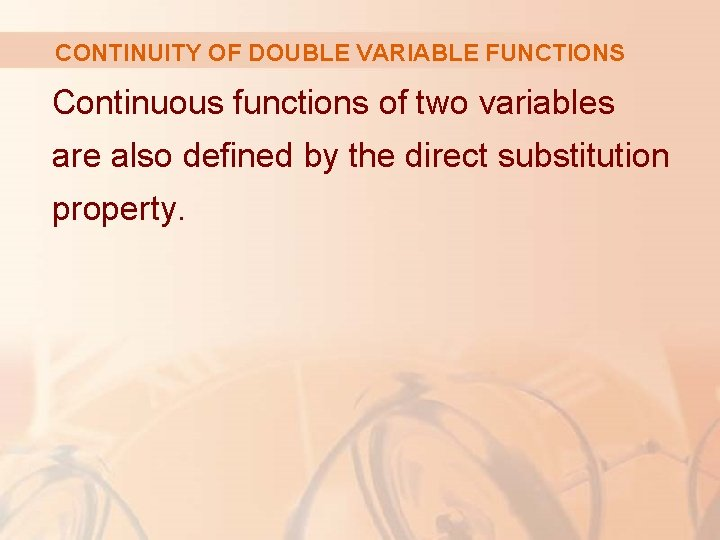CONTINUITY OF DOUBLE VARIABLE FUNCTIONS Continuous functions of two variables are also defined by the direct substitution property.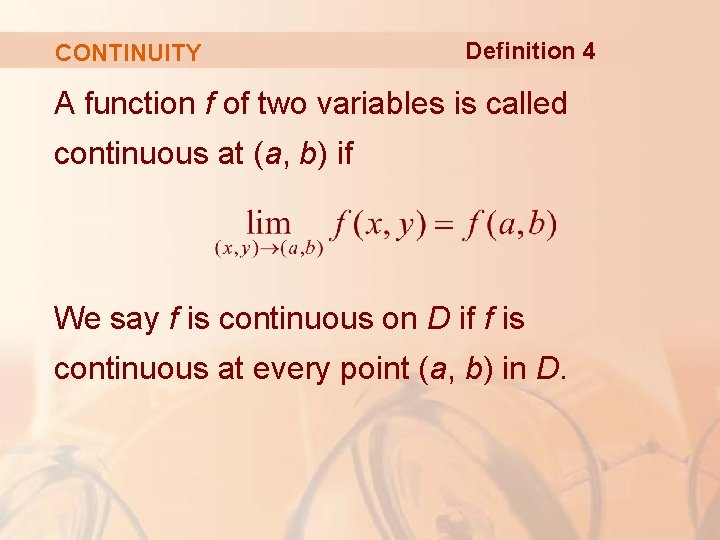CONTINUITY Definition 4 A function f of two variables is called continuous at (a, b) if We say f is continuous on D if f is continuous at every point (a, b) in D.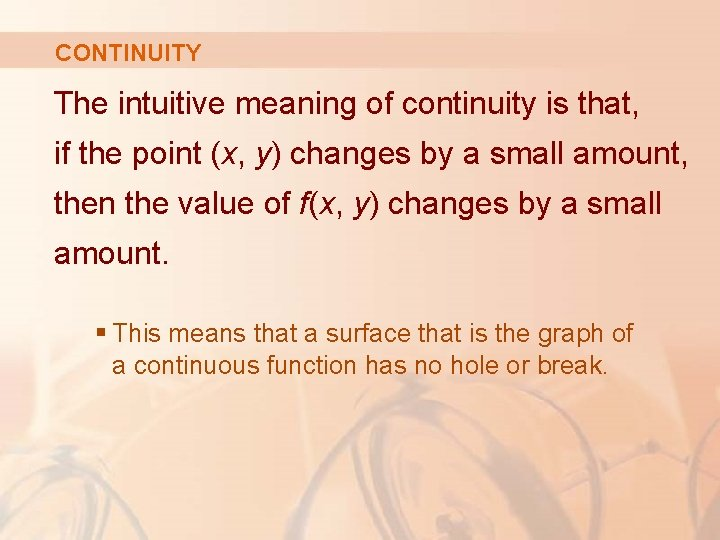CONTINUITY The intuitive meaning of continuity is that, if the point (x, y) changes by a small amount, then the value of f(x, y) changes by a small amount. § This means that a surface that is the graph of a continuous function has no hole or break.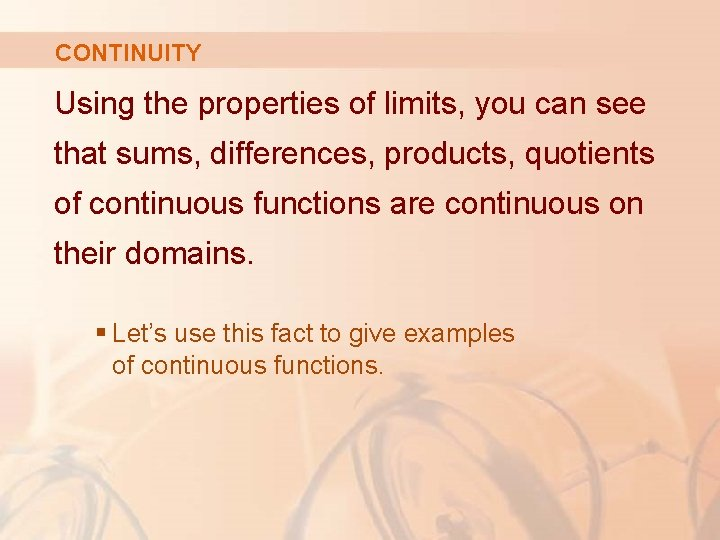CONTINUITY Using the properties of limits, you can see that sums, differences, products, quotients of continuous functions are continuous on their domains. § Let’s use this fact to give examples of continuous functions.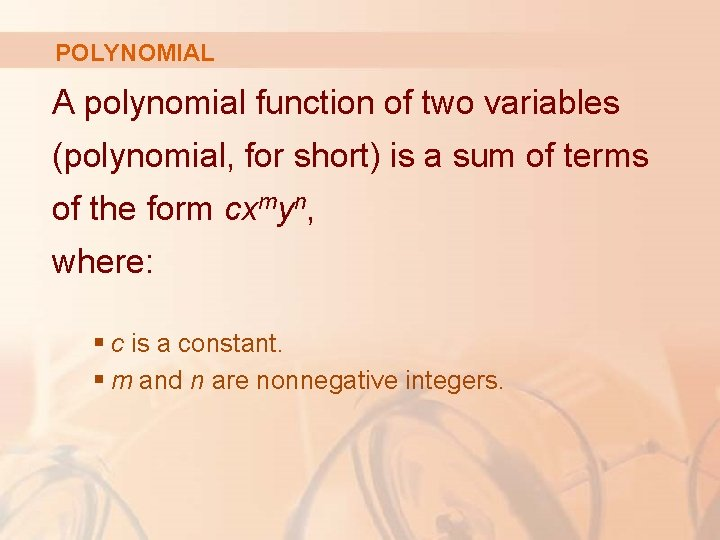POLYNOMIAL A polynomial function of two variables (polynomial, for short) is a sum of terms of the form cxmyn, where: § c is a constant. § m and n are nonnegative integers.RATIONAL FUNCTION A rational function is a ratio of polynomials.RATIONAL FUNCTION VS. POLYNOMIAL is a polynomial. is a rational function.CONTINUITY The limits in Equations 2 show that the functions f(x, y) = x, g(x, y) = y, h(x, y) = c are continuous.CONTINUOUS POLYNOMIALS Any polynomial can be built up out of the simple functions f, g, and h by multiplication and addition. § It follows that all polynomials are continuous on R 2.CONTINUOUS RATIONAL FUNCTIONS Likewise, any rational function is continuous on its domain because it is a quotient of continuous functions.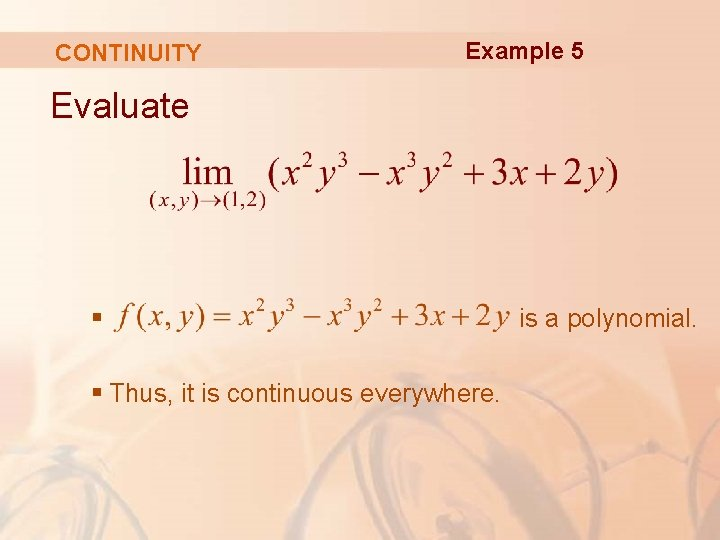CONTINUITY Example 5 Evaluate § § Thus, it is continuous everywhere. is a polynomial.CONTINUITY Example 5 § Hence, we can find the limit by direct substitution:CONTINUITY Where is the function continuous? Example 6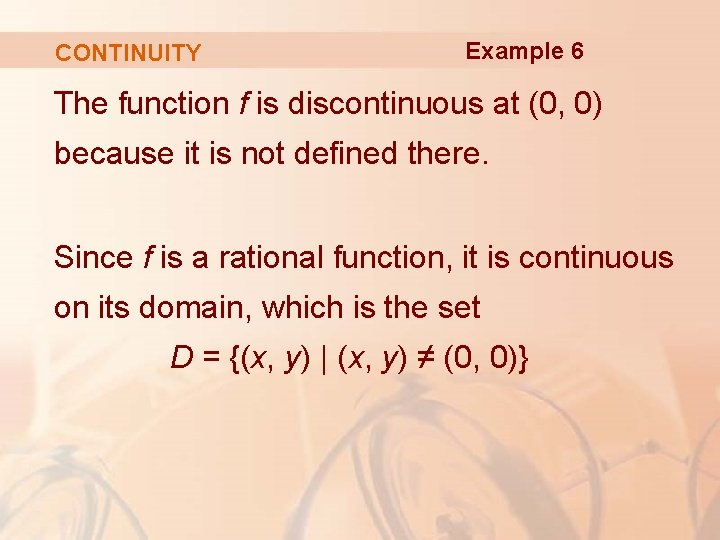CONTINUITY Example 6 The function f is discontinuous at (0, 0) because it is not defined there. Since f is a rational function, it is continuous on its domain, which is the set D = {(x, y) | (x, y) ≠ (0, 0)}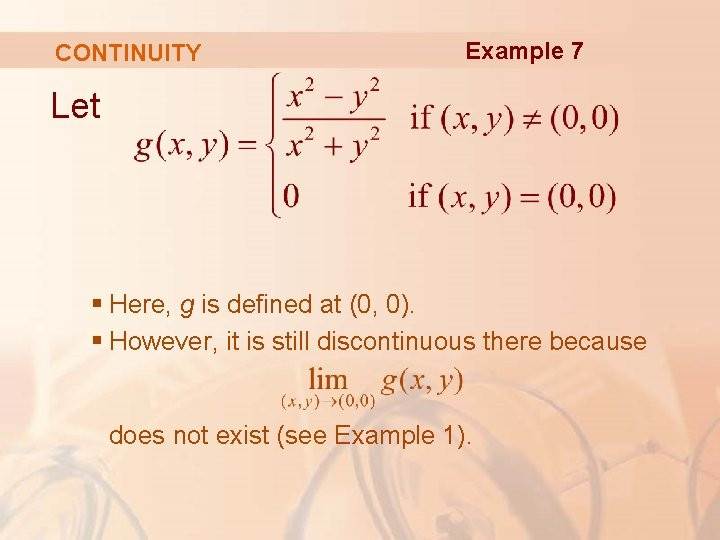CONTINUITY Example 7 Let § Here, g is defined at (0, 0). § However, it is still discontinuous there because does not exist (see Example 1).CONTINUITY Let Example 8CONTINUITY Example 8 We know f is continuous for (x, y) ≠ (0, 0) since it is equal to a rational function there. Also, from Example 4, we have: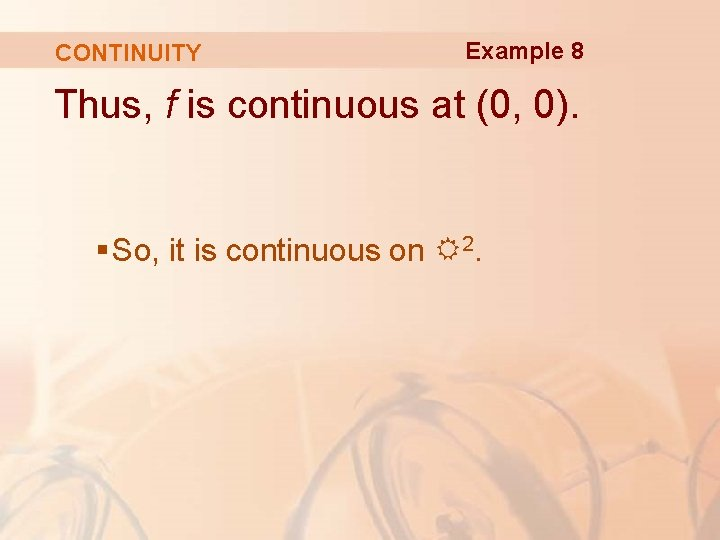CONTINUITY Example 8 Thus, f is continuous at (0, 0). § So, it is continuous on R 2.CONTINUITY This figure shows the graph of the continuous function in Example 8.COMPOSITE FUNCTIONS Just as for functions of one variable, composition is another way of combining two continuous functions to get a third.COMPOSITE FUNCTIONS In fact, it can be shown that, if f is a continuous function of two variables and g is a continuous function of a single variable defined on the range of f, then § The composite function h = g ◦ f defined by h(x, y) = g(f(x, y)) is also a continuous function.COMPOSITE FUNCTIONS Example 9 Where is the function h(x, y) = arctan(y/x) continuous? § The function f(x, y) = y/x is a rational function and therefore continuous except on the line x = 0. § The function g(t) = arctan t is continuous everywhere.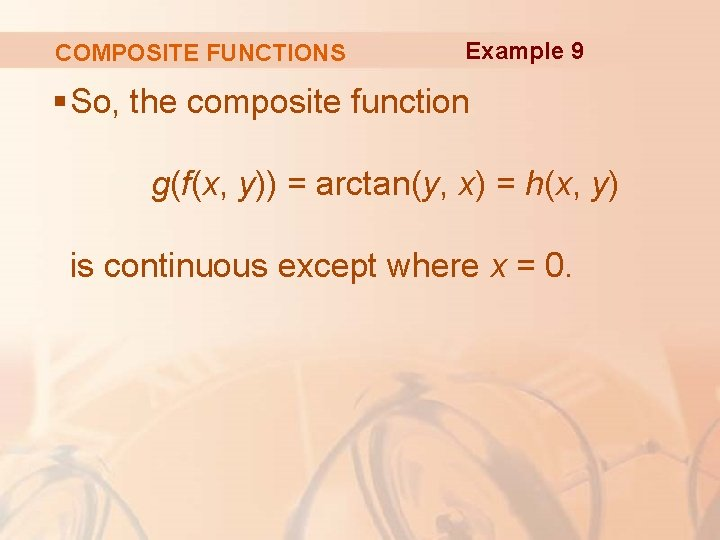COMPOSITE FUNCTIONS Example 9 § So, the composite function g(f(x, y)) = arctan(y, x) = h(x, y) is continuous except where x = 0.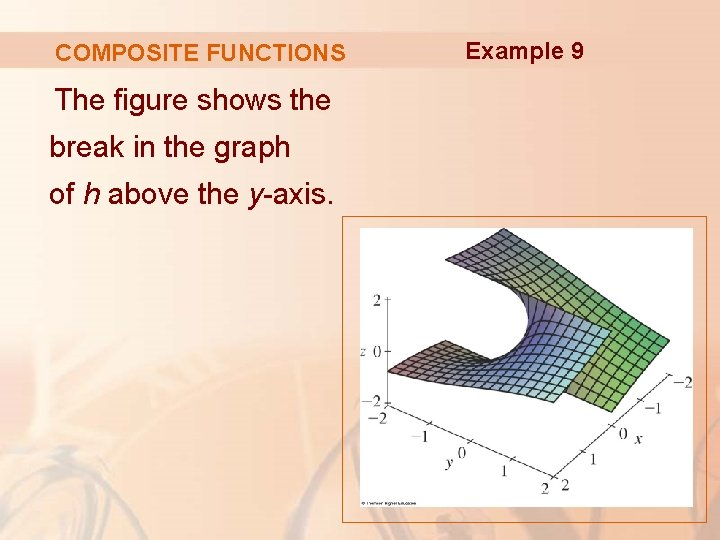COMPOSITE FUNCTIONS The figure shows the break in the graph of h above the y-axis. Example 9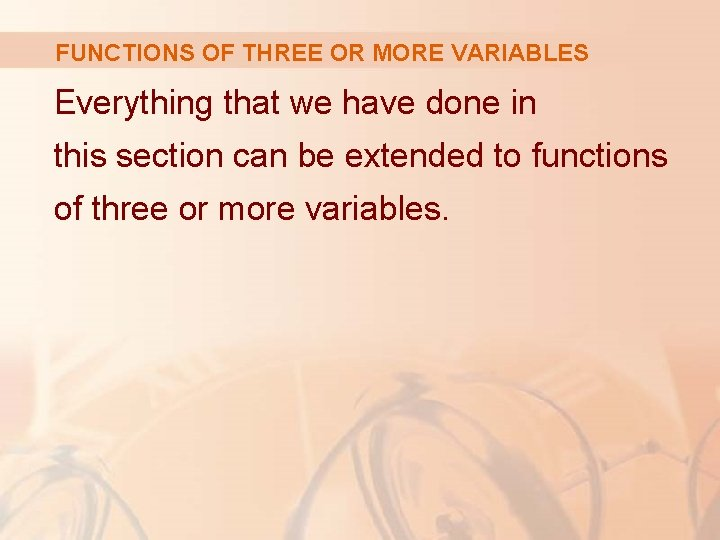FUNCTIONS OF THREE OR MORE VARIABLES Everything that we have done in this section can be extended to functions of three or more variables.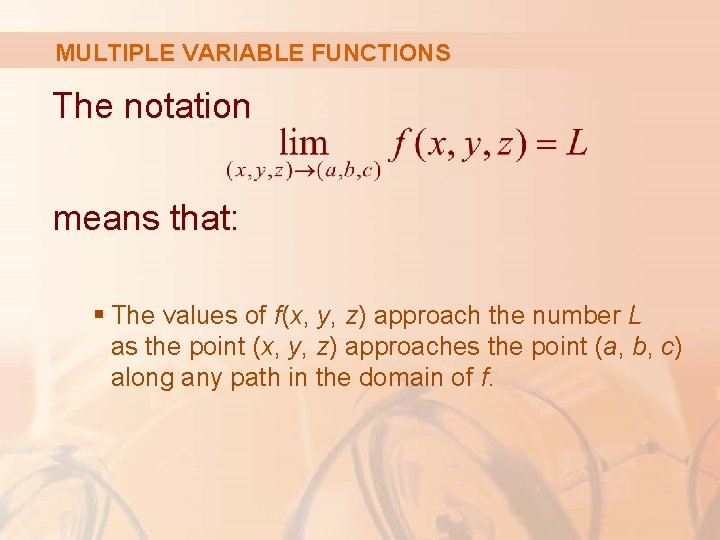MULTIPLE VARIABLE FUNCTIONS The notation means that: § The values of f(x, y, z) approach the number L as the point (x, y, z) approaches the point (a, b, c) along any path in the domain of f.MULTIPLE VARIABLE FUNCTIONS The distance between two points (x, y, z) and (a, b, c) in R 3 is given by: § Thus, we can write the precise definition as follows.MULTIPLE VARIABLE FUNCTIONS For every number ε > 0, there is a corresponding number δ > 0 such that, if (x, y, z) is in the domain of f and then |f(x, y, z) – L| < εMULTIPLE VARIABLE FUNCTIONS The function f is continuous at (a, b, c) if: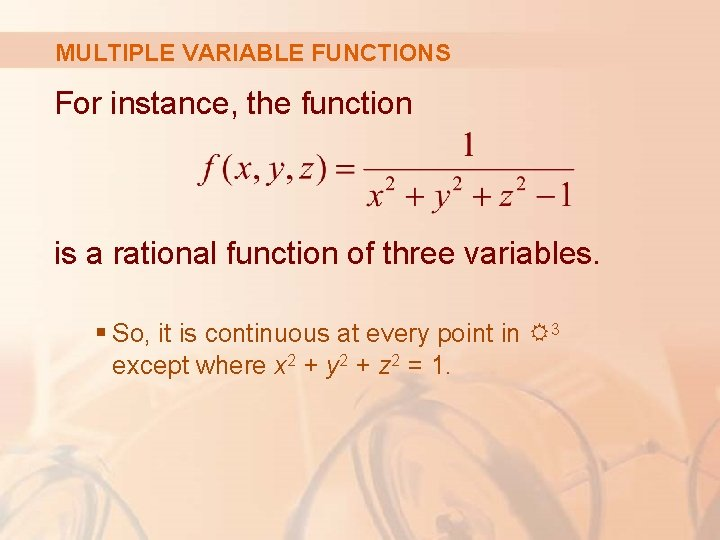MULTIPLE VARIABLE FUNCTIONS For instance, the function is a rational function of three variables. § So, it is continuous at every point in R 3 except where x 2 + y 2 + z 2 = 1.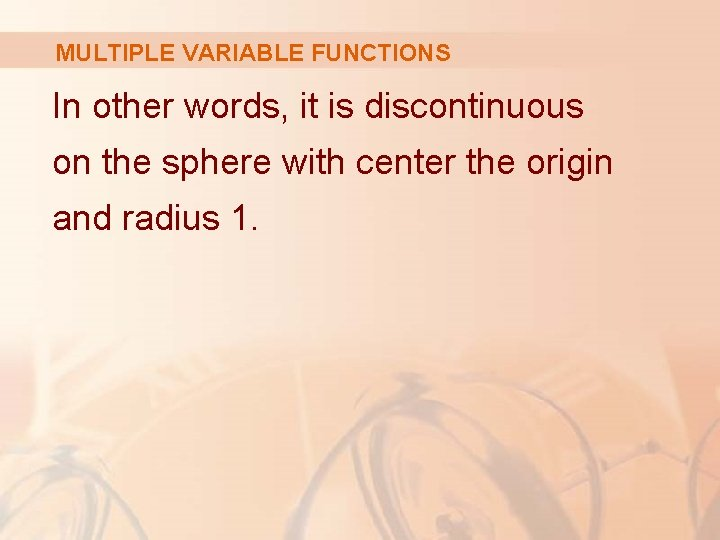MULTIPLE VARIABLE FUNCTIONS In other words, it is discontinuous on the sphere with center the origin and radius 1.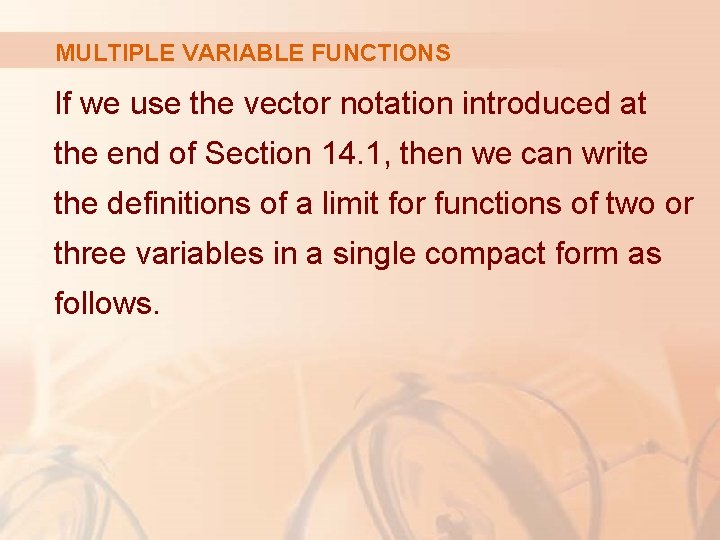MULTIPLE VARIABLE FUNCTIONS If we use the vector notation introduced at the end of Section 14. 1, then we can write the definitions of a limit for functions of two or three variables in a single compact form as follows.MULTIPLE VARIABLE FUNCTIONS Equation 5 If f is defined on a subset D of Rn, then means that, for every number ε > 0, there is a corresponding number δ > 0 such thatMULTIPLE VARIABLE FUNCTIONS If n = 1, then x=x and a=a § So, Equation 5 is just the definition of a limit for functions of a single variable.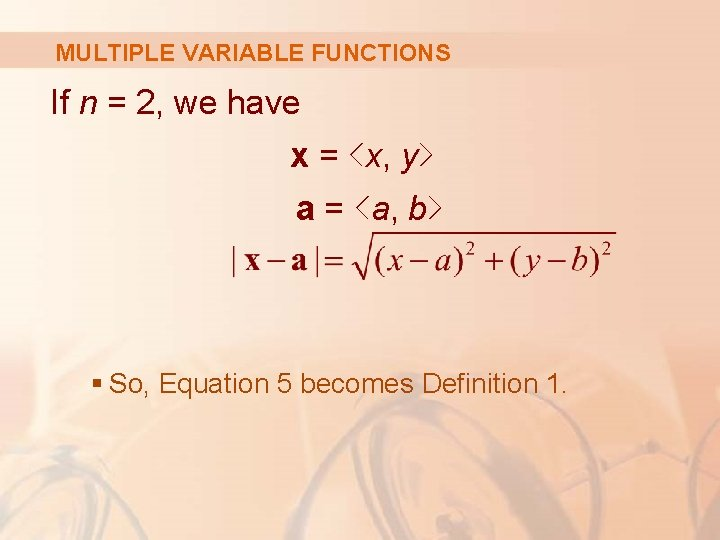MULTIPLE VARIABLE FUNCTIONS If n = 2, we have x = <x, y> a = <a, b> § So, Equation 5 becomes Definition 1.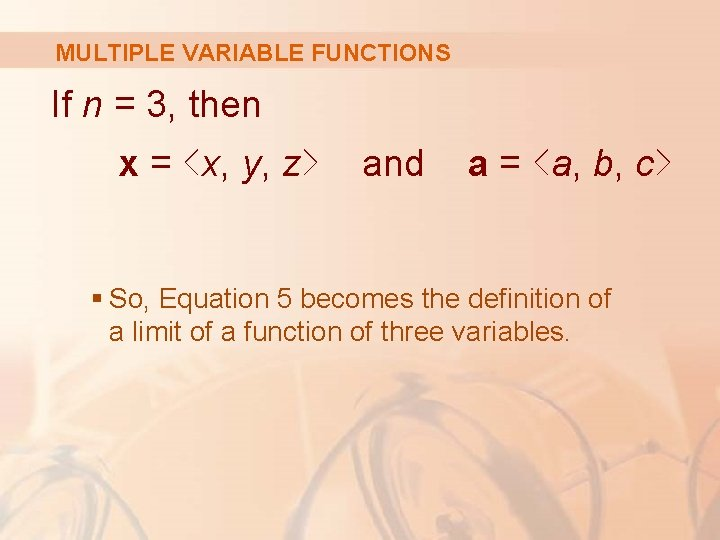MULTIPLE VARIABLE FUNCTIONS If n = 3, then x = <x, y, z> and a = <a, b, c> § So, Equation 5 becomes the definition of a limit of a function of three variables.MULTIPLE VARIABLE FUNCTIONS In each case, the definition of continuity can be written as: